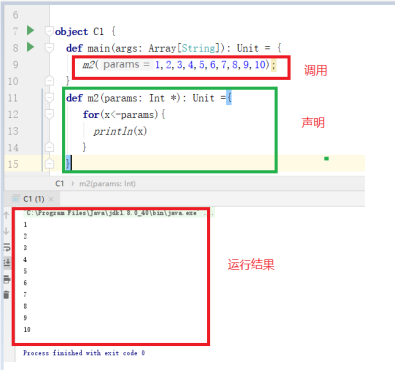# scala基础语法-----Spark基础

scala基本语法

https://www.runoob.com/scala/scala-tutorial.htm

# 1.变量声明

/**

* 定义变量使用var或者val关 键 字

* 语法:

*  var | val 变量名称(: 数据类型) =变量值  */

// 使用val修饰的变量, 值不能为修改,相当于java中final修饰的变量

val name = "tom"

name=”李四”  //错误

// 使用var修饰的变量,值可以修改

var age = 18

age=40  //正确  ，可以修改

// 定义变量时,可以指定数据类型,也可以不指定,不指定时编译器会自动推测变量的数据类型

val name2 : String = "jack"

# 2.变量声明字符串的格式化输出

valal name = "JackMa"
val price = 998.88d
val url = "www.baidu.com"
// 普通输出,注意这里是可以使用逗号分隔的，但是在java中，我们是需要用“+”号拼接
println("name=" + name,"price="+price,"url="+url)
// 'f'插值器允许创建一个格式化的字符串，类似于C语言中的printf。
// 在使用'f'插值器时，所有变量引用都应该是printf样式格式说明符，如％d ,％i ,％f等 。
// 这里$name％s打印String变量name, println(f"姓名：$name%s,价格：$price%1.3f,网址：$url%s")
println(f"姓名：$name,价格：$price,网址：$url") // 's'插值器允许在字符串中直接使用变量 // 下列语句中将String型变量（$name）插入到普通字符串中
println(s"name=$name,price=$price,url=$url") //'s'插值器还可以处理任意形式的表达式 val m=12; val n=33; println(s"m+n=${m+n}")

# 4.循环语句

//  定一个数组
var arr = Array(1, 2, 3, 4, 5, 6)
//遍历数组中么个元素
for (ele <- arr) {
print(ele+" ")
} /*output:1 2 3 4 5 6*/
println("")
// 0 to 5 =>会生成一个范围集合Range（0,1,2,3,4,5),左闭右闭
for (i <- 0 to 5) {
print(i+" ") /*output:0,1,2,3,4,5*/
}
println("")
// 0 until 5 =>会生成一个范围集合Range（0,1,2,3,4)，左闭右开
for (i <- 0 until 5) {
print(i+" ")/*output:0,1,2,3,4*/
}
println("")
// for循环中可以增加守卫，下面这段语句是打印arr数组中的偶数
for (i <- arr if i % 2 == 0) {
print(i+" ")
} /*input:2,4,6*/
println("\n********************双层for循环****************************")
//双层for循环
for (i <- 1 to 3; j <- 1 to 3 if i != j) {
print(i * 10 + j + "  ")
} /*output:12,13,21,23,31,32*/

for (i <- 1 to 3) {
for(j <- 1 to 3 if i != j)
{
println(i * 10 + j + "")
}
}

1 2 3 4 5 6

0 1 2 3 4 5

0 1 2 3 4

2 4 6

********************双层for循环****************************

12  13  21  23  31  32  12

13

21

23

31

32

********************yield 关键字将满足条件的e的值又组合成一个数组****************************

2

4

6

# 4运算符和运算符重载

Scala 中的+-*/%等操作符的作用与 Java 一样，位操作符 &|^>><<也一样。

a+b

a.+(b)

a 方法 b 可以写成 a.方法(b)

var a=4;
var b=5;
var c=a+b;
var d=a.+(b);
println(c)  //输出是9
println(d)  //输出是9

# 4方法的定义与调用

//方法声明使用关键字def .
def f1(): Unit =
{
//函数体
println("我是方法体")
}
//方法名为f1.  无参数， 返回值类型为Unit，表示没有返回值
def  f2(m:Int,n:Int):Int={
return m+n;
//或者直接写m+n  不用带关键字 return
}

//函数调用   和java相同

f1();
var m=f2(4,5);
println(m)

//方法体比较简单的方法还可以如下声明方式

def sayHello1 = print("Say BB1")
def sayHello2() = print("Say BB2")
sayHello1 // 如果方法没有()调用时不能加()
sayHello2() // 可以省略( ) ,也可以不省略

# 4函数的定义与调用

val m1=  println("我是m1函数")
val m2:(Int,Int)=>Int=(x,y)=>{  x+y }
val m3:(Int,Int)=>Unit=(x,y)=>{  var z=x+y;println(z)}
val m4=(x:Int)=>{x+4;}

1. 声明方式不同
2. 函数可以作为方法的参数

//声明一个参数为函数的方法， 第一个参数是函数名，第二个参数是参数

Func：Int=>Int   前面的Int表示函数的参数Int类型, 后面的Int表示函数的返回值是Int类型
def cal(func:Int=>Int,x: Int): Unit ={
var r= func(x);
println(r)
}

（func:Int参数名称和类型，Int,x: Int 返回值类型和返回值参数）

def f1(x:Int): Int ={
x+10;
}

cal(f1,4);

val f1_m=f1 _;
cal(f1_m,4);

# 5可变参数

//说白了，可变参数就是使用一个数组取接收值

def m2(params: Int *): Unit ={
for(x<-params){
println(x)
}
}object C1 {
def main(args: Array[String]): Unit = {
//    1、基础变量
val name="zhh"
//name="zzz" //错误 val不能修改
var name3="zhh" //var可修改
name3="zzz"
val name2:String="jack"
//    2、变量声明字符串的格式化输出
val age=18
println(f"name2=$name%s,age2=$age%s,address=$address%s") println(f"name3=$name ,age3=$age,address=$address%s")
println(s"name4=$name ,age4=$age,address=$address") var m=100 var n=3 println(s"m/n=${m/n}")
println(s"m/n=\${m*1.0/n}")

var i=3
var res=if (i>5) i
//3、循环遍历
var a1=Array(1,2,3,4,5,6);
for(v <- a1) //<-循环遍历a1 将每次遍历的值赋值给v
{
print(v+" ")
}
println()
a1.foreach(v => print(v+" "))   //v相当于函数参数，print(v+" ")相当于函数体 （相当于匿名函数，，，整体类似于函数function(v）{})
println()

for(i <- 1 to 3)
{
print(i+" ")
}
println()

//    输出数组a1的内容
for(i <- 0 to a1.length-1)
{
print(a1(i)+" ") //用a1(i)表示数组元素
}
println()

for(i <- a1 if i%2 ==0 )
{
print(i+" ") //if i%2 ==0 限制输出条件
}
println()

//双层for循环
for (i <- 1 to 3; j <- 1 to 3 if i != j) {
print(i * 10 + j + " ")
} /*output:12,13,21,23,31,32*/
println()
//     与上面循环等价
for (i <- 1 to 3) {
for(j <- 1 to 3 if i != j)
{
print(i * 10 + j + " ")
}
}
println()
//4、方法
var c2=new C2(); //实例对象
c2.f1();
// 5、函数
//此处为main函数中内容
println("我是main函数的内容")
f2();
m3(3,4)  //带参数的函数需要调用（一般不这样用）

//......(1)显式转换
val f3_hanshu=f3 _;  //显式转换 方法f3转换为函数
cal(f3_hanshu,20);
//.....显式转换结束

//....（2）隐式转换
cal(f3,20);  //20作为f3的参数传给方法f3
//    调用cal方法 .注意这里的f1是方法，可以直接作为函数的参数，
//    系统默认f1转为函数。
//.....隐式转换结束

//（3）可变参数
m2(canshu  =1,3,4,5,2,5);

}

//显式转换，隐式转换。。。。。。。
def f3(m:Int): Int ={  //f3作为参数传给方法cal,即f3为参数fun1,
return m+10;
}

def cal (fun1: Int=>Int,m:Int) : Unit ={  //func:Int参数名称和类型，Int,x: Int 返回值类型和返回值参数
var t=fun1(m);
println(t)
}
//显式转换结束，隐式转换结束。。。。。

//可变参数..............
def m2(canshu : Int *): Unit ={
for(x<-canshu ){
print(x+" ")
}
println()
}
//说白了，可变参数就是使用一个数组取接收值，func此时相当于一个数组
可变参数结束..............

// 5、函数
//程序会先执行hashu,和hanshu2（val 一个函数相当于已经实例化类似于是个变量） 后执行main函数！！！！！

def f2(): Unit ={
println("我是方法f2")
}

def f1(x:Int,y:Int): Int ={
return x+y;
}

val hanshu=println("我是函数hanshu")
val hanshu2={println("我是函数hanshu2222")}
val m3:(Int,Int)=>Int=(x,y)=>{  //带参函数
println("dsfdfsfsff")
x+y
} //m3是函数名称，(Int,Int)函数的参数类型，Int是返回值类型 (x,y)返回值参数，{  x+y }为返回值

}
    package com.zhh

class C2 {
//   4、方法声明
def f1(): Unit ={
println("我是C2.f1")
var t=f2(n1=3,n2=4) //调用方法f2
println(t)

}

def f2(n1:Int,n2:Int): Int ={
//    不能改变n1的值，可用用另一个变量赋予n1的值
var m=n1;
m=222;
return n1+n2
}

}

我是函数hanshu

m/n=33
m/n=33.333333333333336
1 2 3 4 5 6
1 2 3 4 5 6
1 2 3
1 2 3 4 5 6
2 4 6
12 13 21 23 31 32
12 13 21 23 31 32

7

dsfdfsfsff
30
30
1 3 4 5 2 5 

## 常量和变量

val声明出来的为常量，不能再次赋值；可以省略类型，scala会自动推导。

var声明出来的为变量，可以再次赋值；可以省略类型，scala会自动推导。

var a = "xxx"; 等同于 var a : String = "xxx";

a = "String 2";

val b = 2;

b = 3; // 会出错

val a, b = "xxx"; // a, b的值都是 "xxx"

## scala的常用数据类型：

Byte：内置转换为scala.runtime.RichByte

Char：内置转换为scala.runtime.RichChar

Short：内置转换为scala.runtime.RichShort

Int：内置转换为scala.runtime.RichInt

Long：内置转换为scala.runtime.RichLong

Float：内置转换为scala.runtime.RichFloat

Double：内置转换为scala.runtime.RichDouble

Boolean：内置转换为scala.runtime.RichBoolean

1.toLong，其中1会被转换为RichInt类型的实例。

1.to(5)会产生Range(1, 2, 3, 4, 5)

BigInt：

BigDecimal：

### 字符串：

String：对应于scala.collection.immutable.StringOps

## 类型转换

var b : Long = a.toLong

## 操作符重载

val value = 8 + 5

(8)转换成Int对象，调用Int对象的+方法（方法名为+）。

## Scala方法调用的简略写法

a 方法 b

a.方法(b)

a(b)

a.apply(b)

### 匿名方法：

x, y => x + y 或

+

x, y 表示为参数；=>指向方法体；x + y计算和并返回。

+ 中，第一个“”表示第一个参数，第二个“”表示第二个参数。

"sU".count(x => x.isUpper)

import math._

## 单例对象object

object定义的对象为单例，可以直接使用 对象名.方法(参数) 进行调用，类似java中的static方法。

## Scala doc

http://scala-lang.org/api

01-04237301-042450
01-04401
01-04533
02-11144
01-04556
12-11796
02-1252
01-04454